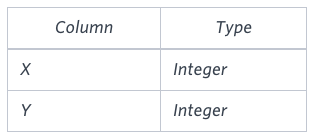## Algorithm

Problem Name: Sql - Symmetric Pairs

In this HackerRank Functions in SQL problem solution,

You are given a table, Functions, containing two columns: and Y.Two pairs (X1, Y1) and (X2, Y2) are said to be symmetric pairs if X1 = Y2 and X2 = Y1.

Write a query to output all such symmetric pairs in ascending order by the value of X. List the rows such that X1 ≤ Y1.

Sample InputSample Output

``````20 20
20 21
22 23
``````

## Code Examples

### #1 Code Example with SQL

```Code - SQL```

``````
with t1 as
(select f.x, f.y, row_number() over(order by x,y) as row_num
from functions f)
select t1.x, t2.x
from t1
join t1 t2 on t1.x = t2.y and t1.y = t2.x and t1.row_num < t2.row_num
where t1.x <= t2.x
order by t1.x;
``````
Copy The Code &